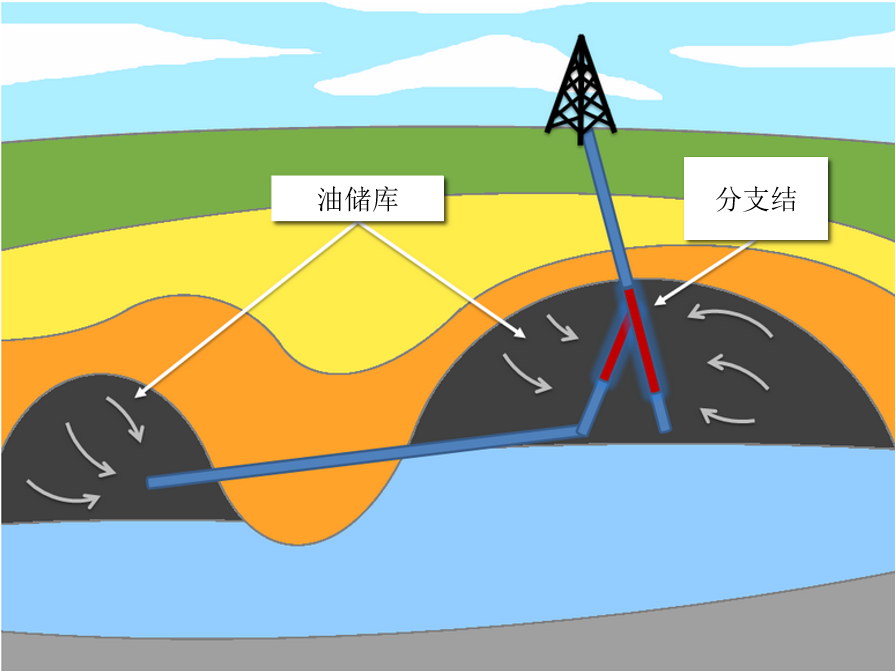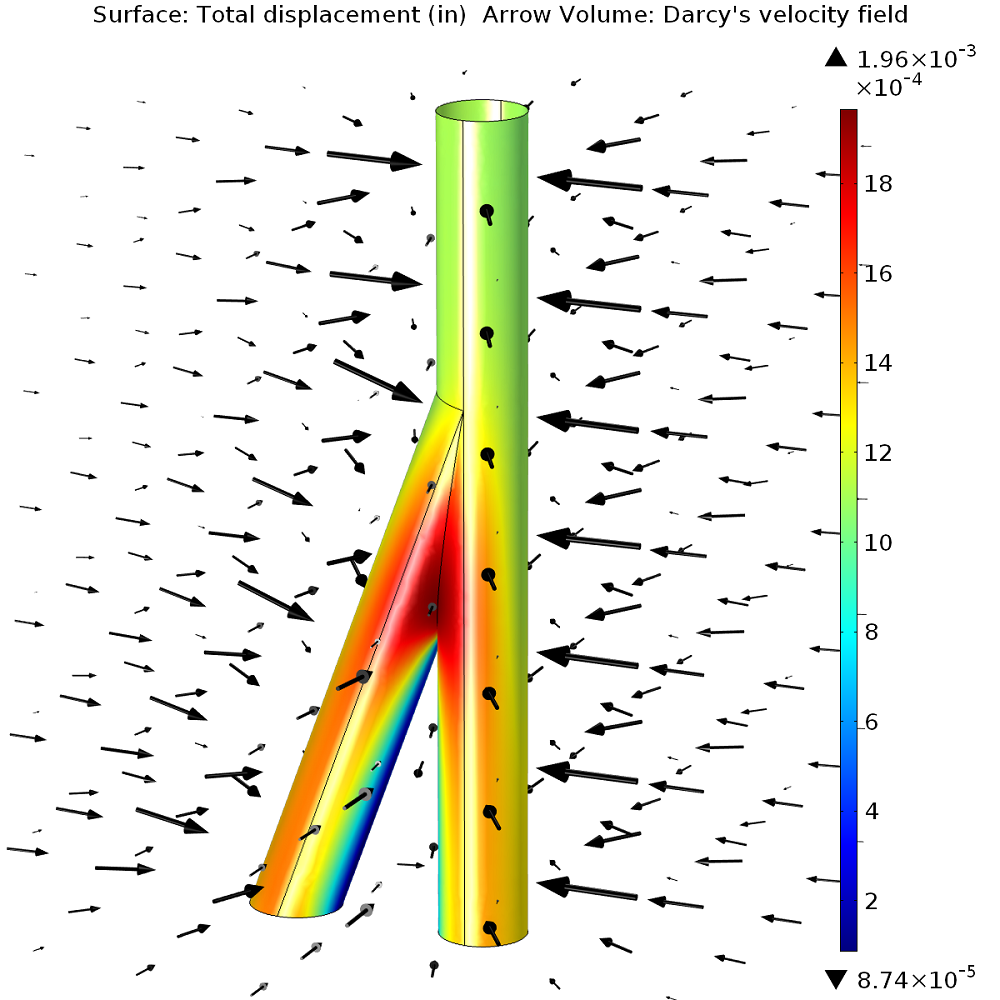# 如何将多分支井的石油开采成本降到最低

2014年 8月 18日

### 多分支井钻井Natural resource recovery：机械泵将石油从储油库运送到地表，邻近的储油库通过与分支结相连的分支井进行开采。

### 多分支井仿真#### 物理方程

\nabla \cdot \left (-\frac{\kappa}{\mu} \nabla p_f \right ) = 0

\sigma = \boldsymbol{C} \epsilon-\alpha_B p_f \boldsymbol{I}

-\nabla \cdot \sigma = \boldsymbol{F}

\rho_f 流体密度

0.0361 lb/in3

\rho_s 固体密度

0.0861 lb/in3

\mu 流体动态粘度

1·10-7 psi·s

\kappa 渗透率

1·10-13 in2

E 杨氏模量

0.43·106 psi

\nu 泊松比

0.16

p_r 油藏压力

122.45 psi

p_w 油井压力

0 psi

#### 仿真结果### 油井稳定性判定

fail=(\sigma_3 +p)-Q(\sigma_1 +p)+N \left ( 1+ \frac{\sigma_2-\sigma_1}{\sigma_3-\sigma_1} \right )

Q=\frac{1+sin\phi}{1-sin\phi},~~~~ N=\frac{2 cos\phi}{1-sin\phi} S_0

S_0 为库伦凝聚力，\phi 为库伦摩擦角。如果 fail > 0 表示油井是稳定的；fail = 0 表示油井壁的岩石已经碎裂；fail < 0 表示油井会出现大面积坍塌。failure 值计算结果，风险最大的位置恰好位于分支结上。

failure值越小，表明失效的风险越大。如前文分析，失效风险最大的地方位于分支结上。如果对油井施加类似套管的支撑力，失效风险将会下降。